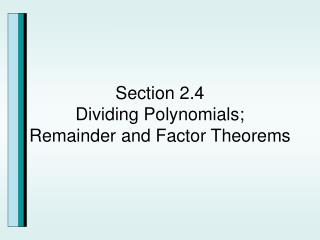DownloadDownload PresentationSection 2.4 Dividing Polynomials; Remainder and Factor Theorems

# Section 2.4 Dividing Polynomials; Remainder and Factor Theorems

Télécharger la présentation## Section 2.4 Dividing Polynomials; Remainder and Factor Theorems

- - - - - - - - - - - - - - - - - - - - - - - - - - - E N D - - - - - - - - - - - - - - - - - - - - - - - - - - -
##### Presentation Transcript

1. Long Division of Polynomials and The Division Algorithm

2. Long Division of Polynomials

3. Long Division of Polynomials

4. Long Division of Polynomials with Missing Terms You need to leave a hole when you have missing terms. This technique will help you line up like terms. See the dividend above.

5. Example Divide using Long Division.

6. Example Divide using Long Division.

7. Dividing Polynomials Using Synthetic Division

8. Comparison of Long Division and Synthetic Division of X3 +4x2-5x+5 divided by x-3

9. Steps of Synthetic Division dividing 5x3+6x+8 by x+2 Put in a 0 for the missing term.

10. Using synthetic division instead of long division. Notice that the divisor has to be a binomial of degree 1 with no coefficients. Thus:

11. Example Divide using synthetic division.

12. The Remainder Theorem

13. If you are given the function f(x)=x3- 4x2+5x+3 and you want to find f(2), then the remainder of this function when divided by x-2 will give you f(2) f(2)=5

14. Example Use synthetic division and the remainder theorem to find the indicated function value.

15. The Factor Theorem

16. Solve the equation 2x3-3x2-11x+6=0 given that 3 is a zero of f(x)=2x3-3x2-11x+6. The factor theorem tells us that x-3 is a factor of f(x). So we will use both synthetic division and long division to show this and to find another factor. Another factor

17. Example Solve the equation 5x2 + 9x – 2=0 given that -2 is a zero of f(x)= 5x2 + 9x - 2

18. Example Solve the equation x3- 5x2 + 9x - 45 = 0 given that 5 is a zero of f(x)= x3- 5x2 + 9x – 45. Consider all complex number solutions.

19. (a) (b) (c) (d)

20. (a) (b) (c) (d)